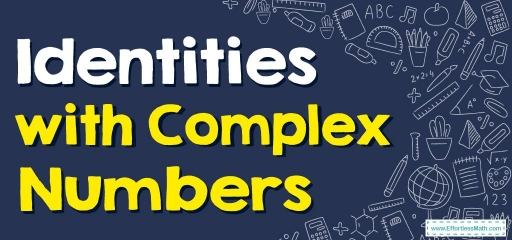# Identities of Complex Numbers

A complex number is a number that is made up of both real and imaginary numbers. Here we talk about some properties of complex numbers. To familiarize more with them read this post.A complex number is written as $$a+ib$$ and usually represented by $$z$$. Where $$a$$ signifies a real number and $$ib$$ represents an imaginary number. In addition, $$a,b$$ are real values, and $$i^2 = -1$$.

As a result, a complex number is a straightforward representation of the addition of two integers, namely a real and an imaginary number. One side is entirely genuine, while the other is entirely imagined.

## A step-by-step guide to identities of complex numbers

The following are some of the properties of complex numbers:

• The sum of two conjugate complex numbers will result in a real number.
• The multiplying of two conjugate complex numbers will produce a real number as well as a complex number.
• If $$x$$ and $$y$$ are real numbers and $$x+yi =0$$, then $$x =0$$ and $$y =0$$ are the same value.
• When two conjugate complex numbers are added together, the result is a real number.
• A real number can be obtained by multiplying two conjugate complex numbers.
• If $$p$$, $$q$$, $$r$$, and $$s$$ are real numbers, then $$p+qi = r+si$$, $$p = r$$, and $$q=s$$.
• The “basic law” of addition and multiplication applies to complex numbers: $$\color{blue}{z_{1 }+ z_{2 }= z_{2 }+ z_{1 } }$$ , $$\color{blue}{z_{1 }. z_{2 }= z_{2 }. z_{1 } }$$
• The complex number follows the “associative law” of addition and multiplication, which is a mathematical rule: $$\color{blue}{(z_{1 }+ z_{2 })+ z_{3 }= z_{1 }+ (z_{2 }+z_{3 })}$$ ,$$\color{blue}{(z_{1 }. z_{2 }).z_{3 }=z_{1 } (z_{2 }. z_{3 } )}$$
• The “distributive law” applies to complex numbers: $$\color{blue}{z_{1 }( z_{2 }+z_{3 })= z_{1 }. z_{2 }+z_{1 }z_{3 } }$$
• In other words, if the sum of two complex numbers is real, and the product of two complex numbers is also genuine, then these complex numbers are conjugated with one another.
• For any two complex numbers, say $$z_{1 }$$ and $$z_{2 }$$, then $$|z_{1 }+z_{2 }|≤|z_{1 }|+|z_{2 }|$$
• When two complex numbers are multiplied by their conjugate value, the output should be a complex number with a positive value.

### Algebraic identities of complex numbers

All algebraic identities apply equally to complex numbers. The addition and subtraction of complex numbers with the exponents of $$2$$ or $$3$$ can be easily solved using algebraic identities of complex numbers.

• $$\color{blue}{(z_{1 }+ z_{2 })^2= (z_{1 })^2+ (z_{2 })^2+2 z_{1 }× z_{2 } }$$
• $$\color{blue}{(z_{1 }- z_{2 })^2= (z_{1 })^2+ (z_{2 })^2-2 z_{1 }× z_{2 } }$$
• $$\color{blue}{(z_{1 })^2- (z_{2 })^2= (z_{1 }+ z_{2 })( z_{1 }- z_{2 }) }$$
• $$\color{blue}{(z_{1 }+ z_{2 })^3= (z_{1 })^3+ 3(z_{1 })^2 z_{2 } +3 (z_{2 })^2 z_{1 }+ (z_{2 })^3 }$$
• $$\color{blue}{(z_{1 }- z_{2 })^3= (z_{1 })^3- 3(z_{1 })^2 z_{2 } +3 (z_{2 })^2 z_{1 }- (z_{2 })^3 }$$

### Identities of Complex Numbers – Example 1:

Find the sum of the complex numbers. $$z_{1 }=-3+i$$ and $$z_{2 }=4-3i$$

$$z_{1 }$$ $$+$$ $$z_{2 }$$ $$=(-3+i)+(4-3i)=(-3+4)+(i-3i)=1-2i$$

### Identities of Complex Numbers – Example 2:

Solve the complex numbers $$(2+i)^2$$.

To solve complex numbers use this formula: $$\color{blue}{(z_{1 }+ z_{2 })^2= (z_{1 })^2+ (z_{2 })^2+2 z_{1 }× z_{2 } }$$

$$(2+i)^2$$ $$=$$ $$(2)^2$$$$+$$$$(i)^2$$$$+(2×2×i)$$$$=4+i^2+4i$$

Then: $$i^2=-1$$ → $$4+i^2=4-1=3$$

Now: $$4+i^2+4i =3+4i$$

### Identities of Complex Numbers – Example 3:

Solve the complex numbers $$(3-i)^3$$.

First, use this formula: $$\color{blue}{(z_{1 }- z_{2 })^3= (z_{1 })^3- 3(z_{1 })^2 z_{2 } +3 (z_{2 })^2 z_{1 }- (z_{2 })^3 }$$

$$(3-i)^3$$ $$=(3)^3-3(3)^2(i)+3(i)^2(3)-(i)^3$$ $$=27-27i+9i^2-i^3$$

Then: $$i^2=-1$$ → $$9i^2=-9$$

$$=27-27i-9-i^3$$ $$=18-27i-i^3$$

$$i^3=-i$$ → $$18-27i-i^3= 18-27i-(-i)=$$ $$18-27i+i$$

Now: $$18-27i+i =18-26i$$

## Exercises for Identities of Complex Numbers

### Simplify.

1. $$\color{blue}{(4+5i)^2}$$
2. $$\color{blue}{(12+5i)+(3+i^2+6i)}$$
3. $$\color{blue}{(20+7i)-(45i+12)}$$
4. $$\color{blue}{(5-4i)^2(3+3i)}$$
5. $$\color{blue}{(i^2-5i)^3}$$
6. $$\color{blue}{(6-i)^2-(10+i)^2}$$
1. $$\color{blue}{-9+40i}$$
2. $$\color{blue}{14+11i}$$
3. $$\color{blue}{8-38i}$$
4. $$\color{blue}{147-93i}$$
5. $$\color{blue}{74+110i}$$
6. $$\color{blue}{-64-32i}$$

### What people say about "Identities of Complex Numbers - Effortless Math: We Help Students Learn to LOVE Mathematics"?

No one replied yet.

X
30% OFF

Limited time only!

Save Over 30%

SAVE $5 It was$16.99 now it is \$11.99